# Mathematician

Mathematician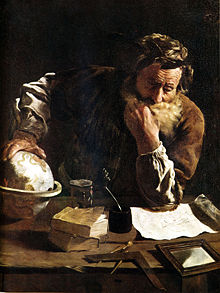Archimedes was among the greatest mathematicians of antiquity.Leonhard Euler is widely considered one of the greatest mathematicians.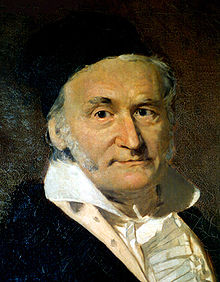Carl Friedrich Gauss was the foremost mathematician of the early 19th century.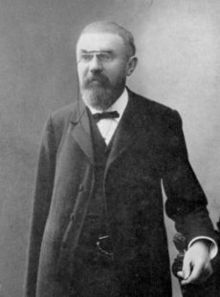Henri Poincaré is considered to be the last mathematician to excel in every field of the mathematics of his time.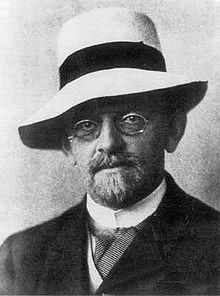David Hilbert was one of the most influential mathematicians of the late 19th and early 20th centuries.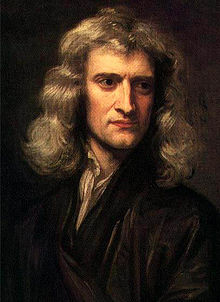Isaac Newton was a pioneering figure in the development of mathematical physics.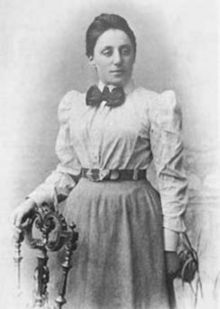Emmy Noether is possibly the most influential female mathematician to date.

A mathematician is a person whose primary area of study is the field of mathematics. Mathematicians are concerned with quantity, structure, space, and change.

Some scientists who research other fields, such as theoretical physics, are also considered mathematicians if their research provides insights into mathematics. Conversely, some mathematicians may provide insights into other fields of research—these people are known as applied mathematicians.

## Education

Mathematicians usually cover breadth of topics within mathematics in their undergraduate education, and then proceed to specialize in topics of their own choice at the graduate level. In some universities, a qualifying exam serves to test both the breadth and depth of a student's understanding of mathematics; the students who pass are permitted to work on a doctoral dissertation. There are notable cases where mathematicians have failed to reflect their ability in their university education, but have nevertheless become remarkable mathematicians. Fermat, for example, is known for having been "Prince of Amateurs", because of his extraordinary achievements with little formal mathematics training.

## Motivation

Mathematicians do research in fields such as logic, set theory, category theory, abstract algebra, number theory, analysis, geometry, topology, dynamical systems, combinatorics, game theory, information theory, numerical analysis, optimization, computation, probability and statistics. These fields comprise both pure mathematics and applied mathematics and establish links between the two. Some fields, such as the theory of dynamical systems, or game theory, are classified as applied mathematics due to the relationships they possess with physics, economics and the other sciences. Whether probability theory and statistics are of theoretical nature, applied nature, or both, is quite controversial among mathematicians. Other branches of mathematics, however, such as logic, number theory, category theory or set theory are accepted to be a part of pure mathematics, although they do indeed find applications in other sciences (predominantly computer science and physics). Likewise, analysis, geometry and topology, although considered pure mathematics, do find applications in theoretical physics - string theory, for instance.

Although it is true that mathematics finds diverse applications in many areas of research, a mathematician does not determine the value of an idea by the diversity of its applications. Mathematics is interesting in its own right, and a majority of mathematicians investigate the diversity of structures studied in mathematics itself. Furthermore, a mathematician is not someone who merely manipulates formulas, numbers or equations—the diversity of mathematics allows for research concerning how concepts in one area of mathematics can be used in other areas too. For instance, if one graphs a set of solutions of an equation in some higher dimensional space, he may ask about the geometric properties of the graph. Thus one can understand equations by a pure understanding of abstract topology or geometry—this idea is of importance in algebraic geometry. Similarly, a mathematician does not restrict his study of numbers to the integers; rather he considers more abstract structures such as rings, and in particular number rings in the context of algebraic number theory. This exemplifies the abstract nature of mathematics and how it is not restricted to questions one may ask in daily life.

In a different direction, mathematicians ask questions about space and transformations, but which are not restricted to geometric figures such as squares and circles. For instance, an active area of research within the field of differential topology concerns itself with the ways in which one can "smooth" higher dimensional figures. In fact, whether one can smooth certain higher dimensional spheres remains open—it is known as the smooth Poincaré conjecture. Another aspect of mathematics, set-theoretic topology and point-set topology, concerns objects of a different nature from objects in our universe, or in a higher dimensional analogue of our universe. These objects behave in a rather strange manner under deformations, and the properties they possess are completely different from those of objects in our universe. For instance, the "distance" between two points on such an object, may depend on the order in which you consider the pair of points. This is quite different from ordinary life, in which it is accepted that the straight line distance from person A to person B is the same as that between person B and person A.

Another aspect of mathematics, often referred to as "foundational mathematics", consists of the fields of logic and set theory. Here, various ideas regarding the ways in which one can prove certain claims are explored. This theory is far more complex than it seems, in that the truth of a claim depends on the context in which the claim is made, unlike basic ideas in daily life where truth is absolute. In fact, although some claims may be true, it is impossible to prove or disprove them in rather natural contexts.

Category theory, another field within "foundational mathematics", is rooted on the abstract axiomatization of the definition of a "class of mathematical structures", referred to as a "category". A category intuitively consists of a collection of objects, and defined relationships between them. While these objects may be anything (such as "tables" or "chairs"), mathematicians are usually interested in particular, more abstract, classes of such objects. In any case, it is the relationships between these objects, and not the actual objects which are predominantly studied.

## Differences with scientists

Mathematics differs from natural sciences in that physical theories in the sciences are tested by experiments, while mathematical statements are supported by proofs that may be verified objectively. If a certain statement is believed to be true by mathematicians (typically because special cases have been confirmed to some degree) but has neither been proved nor disproved, it is called a conjecture, as opposed to the ultimate goal: a theorem that has been proved. Physical theories may be expected to change whenever new information about our physical world is discovered. Mathematics changes in a different way: new ideas do not falsify old ones but rather are used to generalize what was known before to capture a broader range of phenomena. For instance, calculus (in one variable) generalizes to multivariable calculus, which generalizes to analysis on manifolds. The development of algebraic geometry from its classical to modern forms is a particularly striking example of the way an area of mathematics can change radically in its viewpoint without making what was proved before in any way incorrect. While a theorem, once proved, is true forever, our understanding of what the theorem really means gains in profundity as the mathematics around the theorem grows. A mathematician feels that a theorem is better understood when it can be extended to apply in a broader setting than previously known. For instance, Fermat's little theorem for the nonzero integers modulo a prime generalizes to Euler's theorem for the invertible numbers modulo any nonzero integer, which generalizes to Lagrange's theorem for finite groups.

## Women in mathematics

While the majority of mathematicians are male, there have been some demographic changes since World War II. Some prominent female mathematicians are Hypatia of Alexandria (ca. 400 AD), Ada Lovelace (1815–1852), Maria Gaetana Agnesi (1718–1799), Emmy Noether (1882–1935), Sophie Germain (1776–1831), Sofia Kovalevskaya (1850–1891), Alicia Boole Stott (1860–1940), Rózsa Péter (1905–1977), Julia Robinson (1919–1985), Olga Taussky-Todd (1906–1995), Émilie du Châtelet (1706–1749), Mary Cartwright (1900–1998), and Olga Ladyzhenskaya (1922–2004).

The Association for Women in Mathematics is a professional society whose purpose is "to encourage women and girls to study and to have active careers in the mathematical sciences, and to promote equal opportunity and the equal treatment of women and girls in the mathematical sciences." The American Mathematical Society and other mathematical societies offer several prizes aimed at increasing the representation of women and minorities in the future of mathematics.

## Prizes in mathematics

There is no Nobel Prize in mathematics, though sometimes mathematicians have won the Nobel Prize in a different field, such as economics. Prominent prizes in mathematics include the Abel Prize, the Chern Medal, the Fields Medal, the Gauss Prize, the Nemmers Prize, the Balzan Prize, the Crafoord Prize, the Shaw Prize, the Wolf Prize, the Schock Prize, and the Nevanlinna Prize.

## Quotations about mathematicians

The following are quotations about mathematicians, or by mathematicians.

A mathematician is a device for turning coffee into theorems.
—Attributed to both Alfréd Rényi and Paul Erdős
Die Mathematiker sind eine Art Franzosen; redet man mit ihnen, so übersetzen sie es in ihre Sprache, und dann ist es alsobald ganz etwas anderes. (Mathematicians are [like] a sort of Frenchmen; if you talk to them, they translate it into their own language, and then it is immediately something quite different.)
Johann Wolfgang von Goethe
Each generation has its few great mathematicians...and [the others'] research harms no one.
—Alfred W. Adler (1930- ), "Mathematics and Creativity"
In short, I never yet encountered the mere mathematician who could be trusted out of equal roots, or one who did not clandestinely hold it as a point of his faith that x squared + px was absolutely and unconditionally equal to q. Say to one of these gentlemen, by way of experiment, if you please, that you believe occasions may occur where x squared + px is not altogether equal to q, and, having made him understand what you mean, get out of his reach as speedily as convenient, for, beyond doubt, he will endeavor to knock you down.
Edgar Allan Poe, The purloined letter
A mathematician, like a painter or poet, is a maker of patterns. If his patterns are more permanent than theirs, it is because they are made with ideas.
G. H. Hardy, A Mathematician's Apology
Some of you may have met mathematicians and wondered how they got that way.
Tom Lehrer
It is impossible to be a mathematician without being a poet in soul.
Sofia Kovalevskaya

## See also

Some notable mathematicians include Archimedes of Syracuse, Leonhard Euler, Carl Gauss, Johann Bernoulli, Jacob Bernoulli, Aryabhatta, Brahmagupta, Omar Khayyám, Muhammad ibn Mūsā al-Khwārizmī, Bernhard Riemann, Gottfried Leibniz, Andrei Kolmogorov, Euclid of Alexandria, Jules Henri Poincaré, Srinivasa Ramanujan, Alexander Grothendieck, David Hilbert, Alan Turing, von Neumann, Kurt Godel, Joseph-Louis Lagrange, Georg Cantor, William Rowan Hamilton, Carl Jacobi, Évariste Galois, Nikolay Lobachevsky, Rene Descartes, Joseph Fourier, Pierre-Simon Laplace, Robert Lee Moore, Alonzo Church, Nikolay Bogolyubov and Pierre de Fermat.

Wikimedia Foundation. 2010.

### Look at other dictionaries:

• Mathematician — Math e*ma*ti cian, n. [Cf. F. math[ e]maticien.] One versed in mathematics. [1913 Webster] …   The Collaborative International Dictionary of English

• mathematician — (n.) early 15c., from M.Fr. mathematicien, from mathematique, from L. mathematicus (see MATHEMATIC (Cf. mathematic)) …   Etymology dictionary

• mathematician — [math΄ə mə tish′ən, math΄mətish′ən] n. [ME mathematicion < MFr mathematicien] an expert or specialist in mathematics …   English World dictionary

• mathematician — [[t]mæ̱θəmətɪ̱ʃ(ə)n[/t]] mathematicians 1) N COUNT A mathematician is a person who is trained in the study of numbers and calculations. The risks can be so complex that banks hire mathematicians to puzzle them out. 2) N COUNT A mathematician is a …   English dictionary

• mathematician — UK [ˌmæθ(ə)məˈtɪʃ(ə)n] / US noun [countable] Word forms mathematician : singular mathematician plural mathematicians someone who studies or teaches mathematics …   English dictionary

• mathematician — mathematics ► PLURAL NOUN (usu. treated as sing. ) ▪ the branch of science concerned with number, quantity, and space, either as abstract ideas (pure mathematics) or as applied to physics, engineering, and other subjects (applied mathematics).… …   English terms dictionary

• mathematician — noun Date: 15th century a specialist or expert in mathematics …   New Collegiate Dictionary

• mathematician — /math euh meuh tish euhn/, n. an expert or specialist in mathematics. [1400 50; late ME mathematicion. See MATHEMATICS, IAN] * * * …   Universalium

• mathematician — noun /ˈmæθ(.ə)ˌmə.tɪʃ.ən/ An expert on mathematics …   Wiktionary

• mathematician — noun ADJECTIVE ▪ brilliant ▪ distinguished, eminent, great, noted ▪ one of the greatest mathematicians of all time ▪ applied …   Collocations dictionary

### Share the article and excerpts

##### Direct link
Do a right-click on the link above
and select “Copy Link”